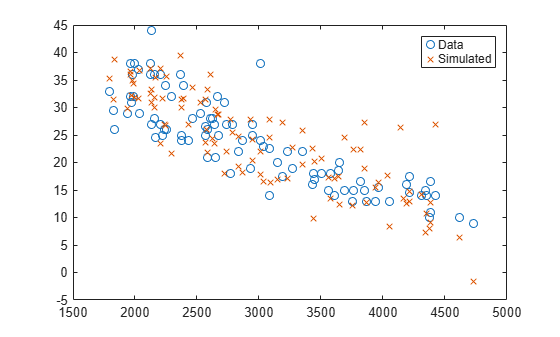Documentation

# random

Simulate responses with random noise for linear regression model

## Syntax

``ysim = random(mdl,Xnew)``

## Description

example

````ysim = random(mdl,Xnew)` simulates responses to the predictor data in `Xnew` using the linear model `mdl`, adding random noise.```

## Examples

collapse all

Create a quadratic model of car mileage as a function of weight from the `carsmall` data set.

```load carsmall X = Weight; y = MPG; mdl = fitlm(X,y,'quadratic');```

Create simulated responses to the data with random noise.

`ysim = random(mdl,X);`

Plot the original responses and the simulated responses to see how they differ.

```plot(X,y,'o',X,ysim,'x') legend('Data','Simulated')```## Input Arguments

collapse all

Linear regression model object, specified as a `LinearModel` object created by using `fitlm` or `stepwiselm`, or a `CompactLinearModel` object created by using `compact`.

New predictor input values, specified as a table, dataset array, or matrix. Each row of `Xnew` corresponds to one observation, and each column corresponds to one variable.

• If `Xnew` is a table or dataset array, it must contain predictors that have the same predictor names as in the `PredictorNames` property of `mdl`.

• If `Xnew` is a matrix, it must have the same number of variables (columns) in the same order as the predictor input used to create `mdl`. Note that `Xnew` must also contain any predictor variables that are not used as predictors in the fitted model. Also, all variables used in creating `mdl` must be numeric. To treat numerical predictors as categorical, identify the predictors using the `'CategoricalVars'` name-value pair argument when you create `mdl`.

Data Types: `single` | `double` | `table`

## Output Arguments

collapse all

Simulated response value, returned as a numeric vector. The simulated value is the predicted response values at `Xnew` perturbed by random noise. The noise is independent and normally distributed, with mean equal to zero and variance equal to the estimated error variance of the model.

## Alternative Functionality

For predictions without random noise, use `predict` or `feval`. These two functions give the same predictions.

• `predict` uses a single input argument with one observation in each row, and gives confidence intervals on its predictions.

• `feval` uses multiple input arguments with one input for each predictor variable.